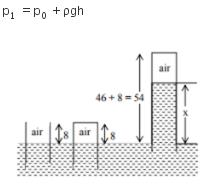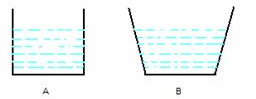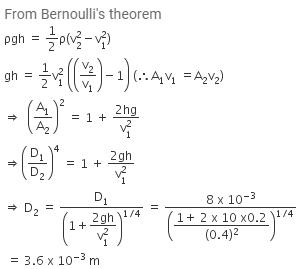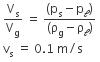Courses

# Test: Pressure and its Applications

## 10 Questions MCQ Test Physics For JEE | Test: Pressure and its Applications

Description
This mock test of Test: Pressure and its Applications for JEE helps you for every JEE entrance exam. This contains 10 Multiple Choice Questions for JEE Test: Pressure and its Applications (mcq) to study with solutions a complete question bank. The solved questions answers in this Test: Pressure and its Applications quiz give you a good mix of easy questions and tough questions. JEE students definitely take this Test: Pressure and its Applications exercise for a better result in the exam. You can find other Test: Pressure and its Applications extra questions, long questions & short questions for JEE on EduRev as well by searching above.
QUESTION: 1

### The pressure of 1 atm = ______ Pa

Solution:

Pressure of 1 atm = 1.013 x 105 Pa

QUESTION: 2

### In a car lift, compressed air exerts a force F1​ on a small piston having a radius of 5cm. This pressure is transmitted to the second piston of a radius of 15cm. If the mass of the car to be lifted is 1350 kg. What is F1​?

Solution:

From Pascal’s law: P​= P2

⇒ F1/A​​= ​F2/A2​​
⇒ F1/πr1​2​ ​= F2/πr2​​
⇒ F1 = (F* r1​2 ) / r22  = 1350 * 9.8 * (5 * 10-2)2 / (15 * 10-2)2 = 1470 N ​= 1.47 * 10N

QUESTION: 3

### Which of the following is not an application of Pascal’s Law?

Solution:

Pascal's law states that the magnitude of pressure within the fluid is equal in all parts.

Option A: Brahma's press is a Hydraulic Press that works on the principle of Pascal's law.

Option B: Submarines don't work on the principle of Pascal's law.

Option C: Hydraulic lifts works on the principle of pascals law i.e. a force applied on a smaller cylinder is transmitted to lift heavy loads using larger cylinders.

Hence, option B is correct.

QUESTION: 4

The formula used to find the pressure on a swimmer h meters below the surface of a lake is: (where Pa is the atmospheric pressure)

Solution:

We know that the pressure at some point inside the water can be represented by: Pa + ρhg
where,
ρ = Density of the liquid
Pa = Atmospheric pressure
H = Depth at which the body is present
g = Gravitational acceleration

QUESTION: 5

The pressure at the bottom of a tank containing a liquid does not depend on:

Solution:

Pressure at the bottom of a tank containing liquid is given as P = hρg which is independent of the surface area bottom of the tank.

QUESTION: 6

An open glass tube is immersed in mercury in such a way that a length of 8 cm extends above the mercury level. The open end of the tube is then closed and sealed and the tube is raised vertically up by additional 46 cm. What will be the length of the air column above mercury in the tube now? (Atmospheric pressure = 76 cm of Hg)

Solution:

Since the system is accelerating horizontally such that no component of acceleration in the vertical direction. Hence, the pressure in the vertical direction will remain unaffected.For air trapped in tube, p1V1 = p2V2
p1 = patm = pg76
V= A.8 [ A = area of cross section]
p2 = patm - ρg(54-x) = ρg(22+x)
V = A.x
ρg76 x 8A = ρg (22+x) (Ax)
x2  + 22x - 78 x 8 by solving, x = 16.

QUESTION: 7

Find the density when a liquid 5 m high in a column exerts a pressure of 80 Pa.

Solution:

► Pressure = Density x Gravity x Height = ρgh
⇒  ρ = P/(g*h) = 80 Pa / (9.8 m/s2 x 5 m)
Density = 1.632 kg/m3

QUESTION: 8

Two vessels with equal base and unequal height have water filled to the same height. The force at the base of the vessels is:Solution:

Force at the bases of two vessels will be equal as force depends on height and area. Since it is the same here, force is equal.

QUESTION: 9

Water is flowing continuously from a tap having an internal diameter 8 x 10-3 m. The water velocity as it leaves the tap is 0.4 ms-1. The diameter of the water stream at a distance 2 x 10-1 m below the tap is close to:

Solution:QUESTION: 10

If the terminal speed of a sphere of gold (density = 19.5 kg/m3 ) is 0.2 m/s in a viscous liquid (density = 1.5 kg/m3 ) of the same size in the same liquid.

Solution: